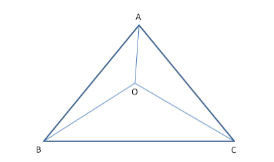# If O is a point within ∆ABC, show that:

Question:

If $O$ is a point within $\triangle A B C$, show that:

(i) $A B+A C>O B+O C$

(ii) $A B+B C+C A>O A+O B+O C$

(iii) $O A+O B+O C>\frac{1}{2}(A B+B C+C A)$

Solution:Given:
In triangle ABC, O is any interior point.
We know that any segment from a point O inside a triangle to any vertex of the triangle cannot be longer than the two sides adjacent to the vertex.
Thus, OA cannot be longer than both AB and CA (if this is possible, then O is outside the triangle).

(i) OA cannot be longer than both AB and CA.

$A B>O B \quad \ldots(1)$

$A C>O C \quad \ldots(2)$

Thus, $A B+A C>O B+O C \quad \ldots[$ Adding (1) and(2) $]$

(ii) $A B>O A \ldots \ldots(3)$

$B C>O B \ldots \ldots(4)$

$C A>O C \ldots \ldots(5)$

Adding the above three equations, we get:

Thus, $A B+B C+C A>O A+O B+O C$       ........(6)

OA cannot be longer than both AB and CA.​

$A B>O B \ldots \ldots(5)$

$A C>O C \ldots \ldots(6)$

$A B+A C>O B+O C \ldots \ldots \ldots[$ On adding (5) and (6) $]$

Thus, the first equation to be proved is shown correct.

(iii) Now, consider the triangles OAC, OBA and OBC.
We have:

$O A+O C>A C$

$O A+O B>A B$

$O B+O C>B C$

Adding the above three equations, we get:

$O A+O C+O A+O B+O B+O C>A B+A C+B C$

$\Rightarrow 2(O A+O B+O C)>A B+A C+B C$

Thus, $O A+O B+O C>\frac{1}{2}(A B+B C+C A)$